# General background

## What is R?

R is a statistical computing language and environment

### What does that mean?

• R is a programming/scripting language
• R is a program that you can use to analyze your data

## Why use R?

### R is free

• R is open source software that you can freely use and modify

### Community

• There is a large community of people using R that you can consult for help
• Mailing lists
• R-help
• R-sig-phylo, R-sig-ecology, R-sig-mixed-models
• Stack Overflow (http://stackoverflow.com)
• Books, manuals, and tutorials
• Introductory Statistics with R - Dalgaard
• Statistics: An Introduction Using R - Crawley
• The R Book - Crawley
• Numerical Ecology With R - Borcard et al.
• Analysis of Phylogenetics and Evolution With R - Paradis

### Huge number of packages

• Anyone can contribute packages of functions to do different statistial analyses
• Over 3895 packages hosted on CRAN
• Pretty much every statistical method has been implemented in R

### Reproducible research

• R's command-line interface makes it easy to keep track of exactly what statistical analyses you have done
• Keep a lab notebook of every command needed to reproduce your analysis

# Working with R

R is a command-line program. The R program includes a command-line environment (the workspace), a text editor, and the option to do a few common tasks using a menu system.

There are several third-party clients that can make working with R easier

## The R workspace

### Basic R Syntax

When you use R, you are working with three types of things:

• operators
• objects
• functions

#### Objects and operators

Open R and type the following commands into the workspace:

x <- 5
x

##  5


You have just created an object named “x”, and used the assignment operator “<-” (pronounced “gets”) to place a value into that object. Typing the name of an object will print the contents of that object.

Object names can be made up of alphanumeric characters but can't start with a number. Object names are case sensitive.

A <- "foo"
a <- "bar"
A

##  "foo"

a

##  "bar"


There are numerous operators in R - arithmetic, logical tests, etc.

x <- 5
y <- 2
z <- x + y
z

##  7

x * y

##  10

x > y

##  TRUE


One thing to note is that the symbol # will cause R to ignore all other input after the # on that line. If you're writing a script to do different analyses, you can use the # symbol to add comments to your script so that you can remember what each line does.

# The next line tests whether z is greater than y
z > y  # everything after the pound sign on this line will be ignored

##  TRUE


#### Functions

Functions are a special type of object that contain instructions on how to do something. If you type the name of a function, the contents of that function will be printed. If you type the name of a function followed by parentheses, that function will be run. Some functions take arguments, others can be run simply by typing their name.

A basic function for interacting with the workspace is ls(), which lists all the objects and functions that you have created in the workspace.

ls()

##  "a" "A" "x" "y" "z"


Another useful function is the help() function. The help function takes an argument - the name of a function or topic you'd like help with - and displays documentation for that topic.

help(ls)  # same as '?ls'



There are several other functions that allow you to get help in R.

help.search("ecology")  # search for a word in all help documentation

RSiteSearch("phylogeny")  # search all R documentation and websites for a topic


There are many functions built in to R. Additionally, you can load sets of functions and data (called packages) to do different types of analysis. We'll talk about packages later.

### Workspace tips

You can save all contents of your workspace using the save.image function. This function takes a filename as an argument. The format of a filename varies depending on your operating system. When working with file names, the file.choose function will prompt you for a filename through an interactive menu.

save.image(file.choose())


If you name your file with a name ending in “.RData”, you can double-click the resulting file to load it back into R. Otherwise, you can load a workspace into R using the load function.

ls()

##  "a" "A" "x" "y" "z"

# what is the current directory?
getwd()

##  "/Users/steve/Dropbox/work/R_workshop_files/introR"

# save workspace image to a file in current directory
save.image("test_workspace.RData")
# delete all objects in the workspace
rm(list = ls())
ls()

## character(0)

# load the workspace we just saved
ls()

##  "a" "A" "x" "y" "z"


#### Tab autocompletion

• If you type part of an object or function name and hit tab, you'll see a list of objects with names matching that text.

#### History

• You can view a history of all the commands you've typed using the history function. Most R environments also let you see the history in a separate window.
history(max.show = 5)  # show the last 5 commands typed

• Most R environments will cycle through your history if you press the up/down arrow

### Best practises for R workflows

One of the advantages of R is that it allows for reproducible research. However, this is only true if you make an effort to be consistent and keep a record of what you have done. This is analogous to a lab or field notebook - you wouldn't do a complicated experiment without keeping a record of what you did. We need to keep similar records while doing statistical analyses. You will need to figure out a workflow that works for you, but here is what I do:

• Every major project/analysis goes in its own directory
• In that directory, there are several files
1. workspace.RData - the R workspace containing all results
2. analysis_scripts.R - a text file with all R commands I ran to get the results
3. results/ - a directory with all the figures and results tables that I generated
• Anyone can replicate my results and figure out exactly how I generated them.

## R Objects

• vector
• matrix
• data.frame
• list

#### vector objects

The simplest type of object in R is a vector. A vector is an object that contains one or more values of the same type. Some common types of vector are:

• numeric - numbers
• character - alphanumeric
• logical - TRUE or FALSE
• factor - ordered or unordered categories

We've already seen how to create a vector (the objects x, y, and z are all numeric vectors). A vector can contain more than one value. To create a vector with more than one value, we use the c function to combine values.

vec.a <- c("first", "second", "third")
vec.a

##  "first"  "second" "third"

vec.b <- c(3, 44, -1.5)
vec.b

##   3.0 44.0 -1.5


We can access subsets of a vector using square brackets.

vec.a

##  "first"

vec.a[2:3]  # same result as vec.a[-1]

##  "second" "third"


A vector can have names for its elements. These names are distinct from the value stored in each element. Names can also be used to access subsets of a vector with square brackets.

vec.c <- c(1, 2, 3)
names(vec.c) <- c("one", "two", "three")
vec.c[c("one", "two")]

## one two
##   1   2


#### matrix objects

A matrix is a two-dimensional numerical array. Similar to vectors, we can access subsets of a matrix using square brackets.

mat.a <- matrix(c(1, 2, 3, 4, 5, 6), nrow = 2, ncol = 3)
mat.a

##      [,1] [,2] [,3]
## [1,]    1    3    5
## [2,]    2    4    6

# subset of a matrix format is [rows, columns]
mat.a[1, ]  # first row

##  1 3 5

mat.a[, 2]  # second column

##  3 4


Matrices may have names for their rows and columns.

rownames(mat.a) <- c("row1", "row2")
colnames(mat.a) <- c("col1", "col2", "col3")
mat.a["row2", 3]

##  6


#### data.frame objects

A data.frame is similar to a matrix, but its columns can be a mixture of different types of data. Most functions that work on a matrix will also work on a data.frame, and we can convert between the two data formats. In addition to square brackets, we can create and access individual columns of a data.frame using the dollar sign.

The data.frame is perhaps the most frequently used type of object for biodiversity analysis since it can contain many different types of data.

df.a <- as.data.frame(mat.a)
df.a$col4 <- c("foo", "bar") dim(df.a)  ##  2 4  df.a  ## col1 col2 col3 col4 ## row1 1 3 5 foo ## row2 2 4 6 bar  df.a$col1  ## same as df.a[,'col1'] or df.a[,1]

##  1 2


#### list objects

A list is a collection of different types of objects. Unlike a matrix or data.frame, the elements of a list do not need to be the same length or type of data. We can access individual elements of a list using the dollar sign, or using double square brackets.

# make a list combining vector, matrix, and data.frame objects
list.a <- list(va = vec.a, vb = vec.b, ma = mat.a, dfa = df.a)
names(list.a)

##  "va"  "vb"  "ma"  "dfa"

# there are several different ways to access elements of the list
list.a$va # same as list.a[['va']] or list.a[]  ##  "first" "second" "third"  ### Data import The easiest way to read your own data sets into R is to first save your data in a text-based spreadsheet format. If you have your data in an Excel spreadsheet (a .xls or .xlsx file), save the spreadsheet in either comma separated (.csv) or tab-delimited text (.txt) format. Then you can read your data into a data.frame in R. mydata <- read.csv(file.choose(), row.names = 1) # comma separated - use column 1 as row names  mydata <- read.table(file.choose(), header = TRUE, sep = "\t") # tab delimited  Similarly, you can write your data to a file that can be opened by your favourite spreadsheet software. If your data are in a data.frame or matrix you can use the write.csv or write.table functions. write.csv(mydata, file.choose())  #### Common pitfalls during data import into R • If a column of your spreadsheet contains anything other than numbers (including spaces), it will be imported as a character. • If there are missing data in your spreadsheet, represent them either as an empty cell, or as the text NA. “NA” is the way that R represents missing data and an NA in your spreadsheet will be read in as a missing value and not a text value. • If you are reading in columns names using the header argument, your column names should not begin with a number and should contain only alphanumeric characters. Any spaces or underscores in your column names will be conveted to a period. ### Data checking and manipulation #### apply We can apply a function to subsets of an object using apply functions. # calculate the sum of each row of a matrix apply(mat.a, MARGIN = 1, FUN = sum)  ## row1 row2 ## 9 12  # calculate the sum of each column of a matrix apply(mat.a, MARGIN = 2, FUN = sum)  ## col1 col2 col3 ## 3 7 11  You can use apply to check whether you've correctly imported your data set. For example, if your data are supposed to be numeric values, you could use the apply function to make sure they've been imported as intended. # check the class of each column of a data.frame sapply(df.a, class)  ## col1 col2 col3 col4 ## "numeric" "numeric" "numeric" "character"  # obtain a summary of each column of a data.frame summary(df.a)  ## col1 col2 col3 col4 ## Min. :1.00 Min. :3.00 Min. :5.00 Length:2 ## 1st Qu.:1.25 1st Qu.:3.25 1st Qu.:5.25 Class :character ## Median :1.50 Median :3.50 Median :5.50 Mode :character ## Mean :1.50 Mean :3.50 Mean :5.50 ## 3rd Qu.:1.75 3rd Qu.:3.75 3rd Qu.:5.75 ## Max. :2.00 Max. :4.00 Max. :6.00  #### reshape Often we record our data in a format that is easy to record, but not ideal for data analysis. There are several functions in R that make it easy to convert among different ways of representing data. # load example data set of plant growth vs. treatment data(PlantGrowth) # the head and tail functions show a subset of the data head(PlantGrowth)  ## weight group ## 1 4.17 ctrl ## 2 5.58 ctrl ## 3 5.18 ctrl ## 4 6.11 ctrl ## 5 4.50 ctrl ## 6 4.61 ctrl  # convert to matrix with weight in rows and group in columns (weight by # group) pg.wide <- unstack(PlantGrowth, weight ~ group) head(pg.wide)  ## ctrl trt1 trt2 ## 1 4.17 4.81 6.31 ## 2 5.58 4.17 5.12 ## 3 5.18 4.41 5.54 ## 4 6.11 3.59 5.50 ## 5 4.50 5.87 5.37 ## 6 4.61 3.83 5.29  # summarize mean weight per group aggregate(PlantGrowth$weight, by = list(group = PlantGrowth$group), mean)  ## group x ## 1 ctrl 5.032 ## 2 trt1 4.661 ## 3 trt2 5.526  There is a R package called plyr (http://plyr.had.co.nz/) that makes these types of data conversions very easy. ### Packages A package is a library of functions and data. #### Finding packages There are more than 3895 R packages available for download from CRAN (!!!). Finding a package to do the analysis you want can be overwhelming. • Use the RSiteSearch function to find a particular method • Use Task Views on CRAN to find packages for different types of analysis • Crantastic (http://crantastic.org/) #### Installing packages Packages can be installed automatically through the workspace. To install the picante package and its dependencies, you would type the following: install.packages("picante", dependencies = TRUE)  #### Using packages Once you have installed a package, you can load it and get a list of the functions and data it contains: library(picante)  ## Loading required package: ape ## Loading required package: vegan ## Loading required package: permute ## Loading required package: lattice ## This is vegan 2.0-9 ## Loading required package: nlme  help(picante) # click on 'Index' at the bottom of help screen for list of functions  By loading the package, you now have access to the functions in that package. Packages can also include data sets, which can be loaded with the data function. # load an example data set from picante data(phylocom) names(phylocom)  ##  "phylo" "sample" "traits"  Packages must include documentation for all functions. Many functions will also include example code, which can be run automatically with the example function. # view the help for the pd function in picante help(pd) # run the example code for the pd function example(pd)  ## ## pd> data(phylocom) ## ## pd> pd(phylocom$sample, phylocom$phylo) ## PD SR ## clump1 16 8 ## clump2a 17 8 ## clump2b 18 8 ## clump4 22 8 ## even 30 8 ## random 27 8  Many packages also include vignettes - these are short documents that include documentation or tutorials on various topics. The following commands will give you a list of vignettes available for all installed packages, and display a vignette from the picante package. vignette()  vignette("picante-intro")  ### Summarizing and visualizing data sets There are numerous functions in R that allow you to summarize data sets. Let's look at an example data set on Iris morphology that is included with R. # load the iris example data set data(iris) head(iris)  ## Sepal.Length Sepal.Width Petal.Length Petal.Width Species ## 1 5.1 3.5 1.4 0.2 setosa ## 2 4.9 3.0 1.4 0.2 setosa ## 3 4.7 3.2 1.3 0.2 setosa ## 4 4.6 3.1 1.5 0.2 setosa ## 5 5.0 3.6 1.4 0.2 setosa ## 6 5.4 3.9 1.7 0.4 setosa  summary(iris)  ## Sepal.Length Sepal.Width Petal.Length Petal.Width ## Min. :4.30 Min. :2.00 Min. :1.00 Min. :0.1 ## 1st Qu.:5.10 1st Qu.:2.80 1st Qu.:1.60 1st Qu.:0.3 ## Median :5.80 Median :3.00 Median :4.35 Median :1.3 ## Mean :5.84 Mean :3.06 Mean :3.76 Mean :1.2 ## 3rd Qu.:6.40 3rd Qu.:3.30 3rd Qu.:5.10 3rd Qu.:1.8 ## Max. :7.90 Max. :4.40 Max. :6.90 Max. :2.5 ## Species ## setosa :50 ## versicolor:50 ## virginica :50 ## ## ##  We can visualize the data using graphics functions built into R. # histogram of sepal width hist(iris$Sepal.Width)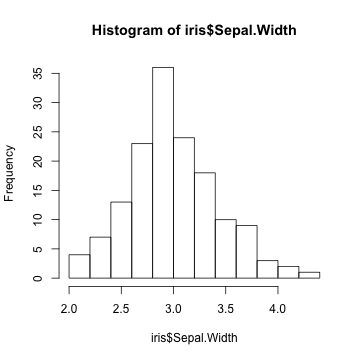# boxplot of petal length by species
boxplot(Petal.Length ~ Species, data = iris, xlab = "Species", ylab = "Petal length")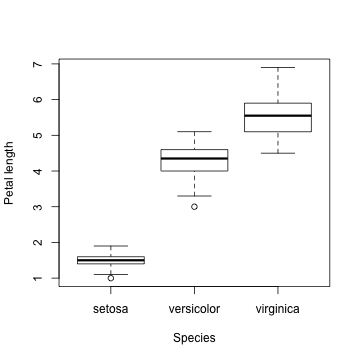# plot relationships among all variables color points by species
pairs(iris[, 1:4], col = iris\$Species)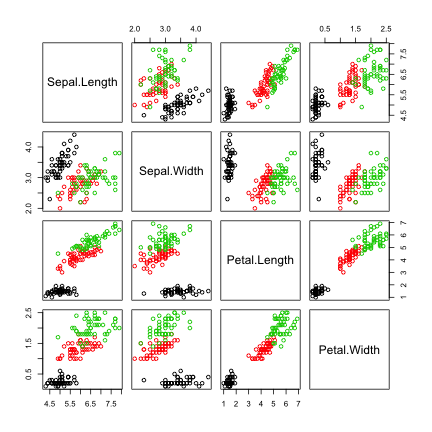We can do some basic statistical analyses of the data. Use a linear model to test whether sepal width differs among species.

# Linear model of sepal width as a function of species
iris.lm <- lm(Sepal.Width ~ Species, data = iris)
# Summarize the linear model
summary(iris.lm)

##
## Call:
## lm(formula = Sepal.Width ~ Species, data = iris)
##
## Residuals:
##    Min     1Q Median     3Q    Max
## -1.128 -0.228  0.026  0.226  0.972
##
## Coefficients:
##                   Estimate Std. Error t value Pr(>|t|)
## (Intercept)         3.4280     0.0480   71.36  < 2e-16 ***
## Speciesversicolor  -0.6580     0.0679   -9.69  < 2e-16 ***
## Speciesvirginica   -0.4540     0.0679   -6.68  4.5e-10 ***
## ---
## Signif. codes:  0 '***' 0.001 '**' 0.01 '*' 0.05 '.' 0.1 ' ' 1
##
## Residual standard error: 0.34 on 147 degrees of freedom
## Multiple R-squared:  0.401,  Adjusted R-squared:  0.393
## F-statistic: 49.2 on 2 and 147 DF,  p-value: <2e-16

# ANOVA test for significance of species effect
anova(iris.lm)

## Analysis of Variance Table
##
## Response: Sepal.Width
##            Df Sum Sq Mean Sq F value Pr(>F)
## Species     2   11.3    5.67    49.2 <2e-16 ***
## Residuals 147   17.0    0.12
## ---
## Signif. codes:  0 '***' 0.001 '**' 0.01 '*' 0.05 '.' 0.1 ' ' 1

# Plot model diagnostics
plot(iris.lm)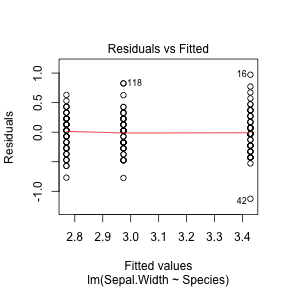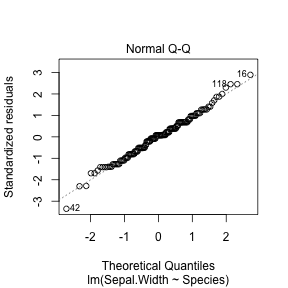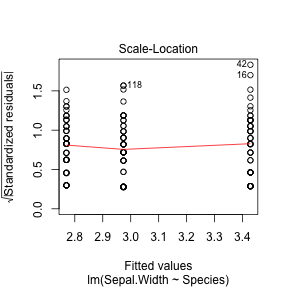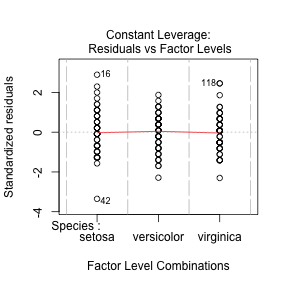# Plot Tukey's honestly significant difference test comparison of
# species-pair differences
plot(TukeyHSD(aov(iris.lm)))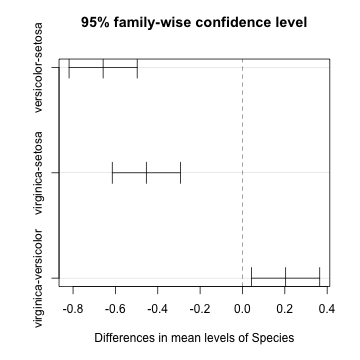#### R Graphics

There are a huge number of graphical functions in R.